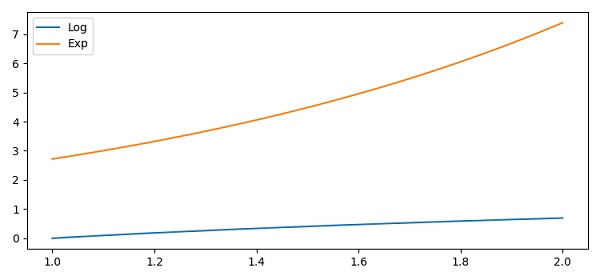# Automatically position text box in Matplotlib

To position a textbox automatically in matplotlib, we can take the following Steps −

• Create xpoints from 1 to 2 and 100 samples.

• Create y1points and y2points using xpoints (Step 1) and numpy.

• Plot xpoints, y1points and y2points using the plot() method.

• To set the label, use the legend() method. It will help to position the text box.

• To display the figure, use the show() method.

## Example

import numpy as np
from matplotlib import pyplot as plt
plt.rcParams["figure.figsize"] = [7.50, 3.50]
plt.rcParams["figure.autolayout"] = True
xpoints = np.linspace(1, 2, 100)
y1points = np.log(xpoints)
y2points = np.exp(xpoints)
plt.plot(xpoints, y1points, label="Log")
plt.plot(xpoints, y2points, label="Exp")
plt.legend()
plt.show()

## Output